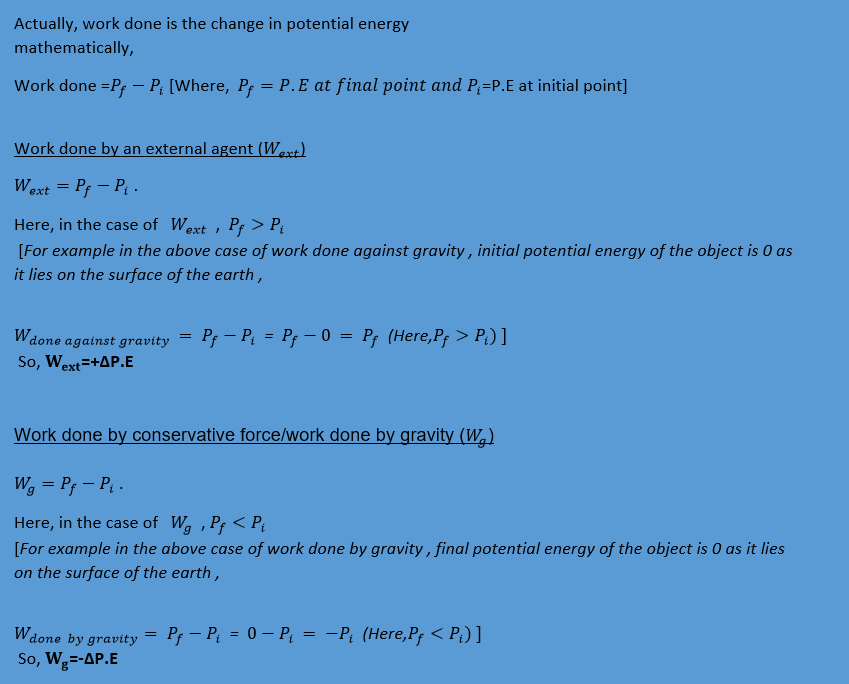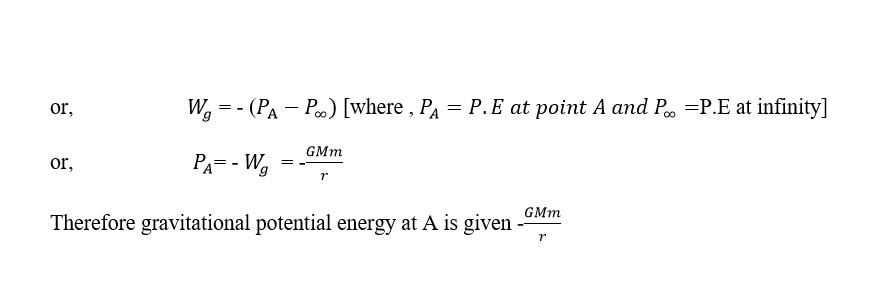Gravitational Potential Energy﻿Analogy to understand G.P.EThe above spring mass system is in equilibrium .If I apply force of F newton to displace it to x meters away from its initial position then

Work done = F * xWhen I stop pulling it further away from x meters  from the initial position , and leave it then it goes back to its initial position .

Who applied the force ? How did it get back to its initial position ?

-The work done by me is  stored as potential energy . When I left pulling it , the potential energy gets converted into the kinetic energy .

Since , I am not a part of spring mass system the work done by me is termed as "work done by an external agent "Let us imagine gravity to work  just like a spring . As , I lift an object to a greater height the spring gets stretched more and more . The work done by me increases with the increment in the stretching of the spring (or the height). Since work done by me is stored as potential energy , we can say the potential energy increases with the height  .

From the above explanation we can create a relationship between work done by me and the potential energy as

Work done by me = Potential energy

﻿If ﻿I stop lifting and gently put it down with a constant velocity then the spring gets compressed. With the compression of spring height decreases. As the height decreases potential energy also decreases . Here , the work done by gravity increases with the decrement in the height . The work done by gravity is positive as  the displacement is towards the direction of force . Work done by me is negative because the displace is opposite to the direction of force applied by me and it keeps the velocity constant throughout the process.
Therefore ,
Work done by gravity(Wg)=- work done by me (Wext)

or,                                     Wg  =-P.E

Note:﻿Definition

The gravitational potential energy at a point in gravitational field is defined as the amount of work done while bringing a body from inf
inity to that point with constant velocity .

infinity - We know that ,  - Wg  = Pf - Pi
To find potential at a point we should assume the initial point to have potential energy  0.
It is assumed that the potential energy at infinity   be  0 .

So ,
-Wg  = Pf - Pinfinity
-Wg  = Pf - 0
-Wg  = P

constant velocity - According to work-energy theorem ,
Work done = change in kinetic energy
If we consider velocity to be constant then change in kinetic energy is 0 .
Here , the work done is only stored as potential energy and is not                                                             converted into kinetic energy. So velocity is considered to be constant .

﻿Derivation